#Function Repository Resource:

Plot a quadric surface, automatically determining the regions of interest, view direction and scaling

Contributed by: Daniel Lichtblau
 ResourceFunction["QuadricPlot3D"][poly] plots the quadric surface given by poly==0. ResourceFunction["QuadricPlot3D"][poly1==poly2] plots the quadric surface given by poly1==poly2. ResourceFunction["QuadricPlot3D"][poly,"ShowCode"] returns the code used to create the plot, wrapped in Hold.

## Examples

### Basic Examples

A hyperboloid of one sheet:

 In:=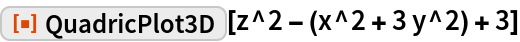Out=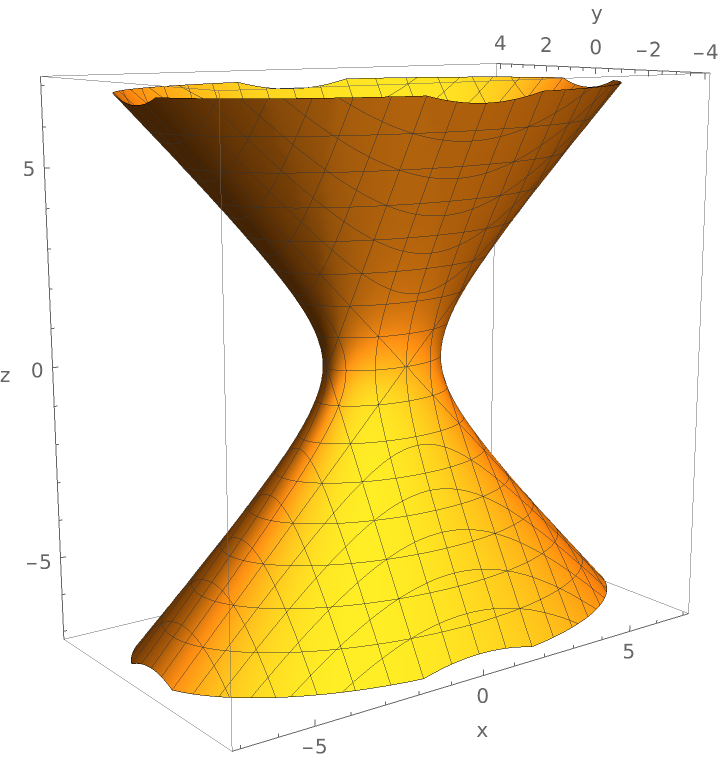A hyperboloid of two sheets, entered in equation form:

 In:=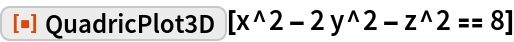Out=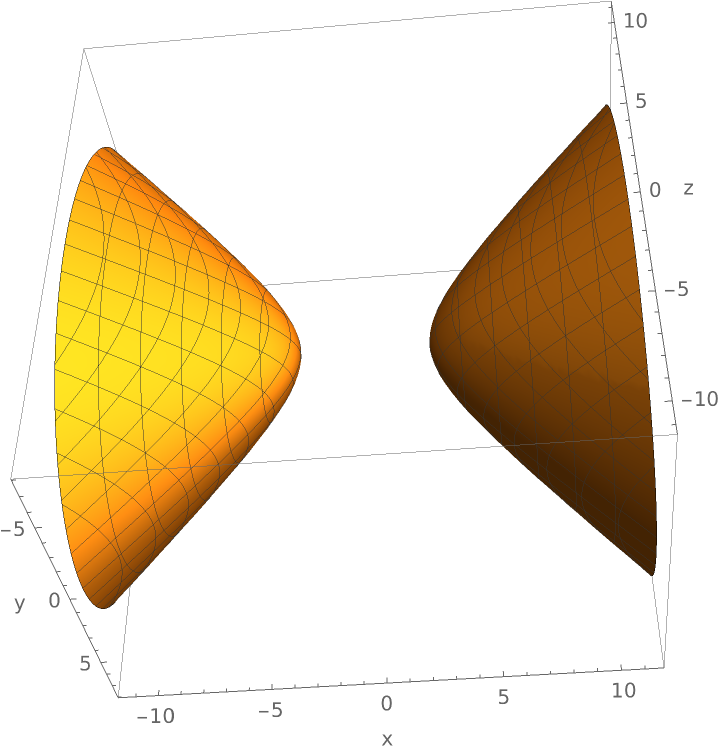Return the code used to create an ellipsoid plot:

 In:=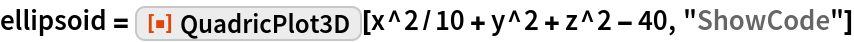Out=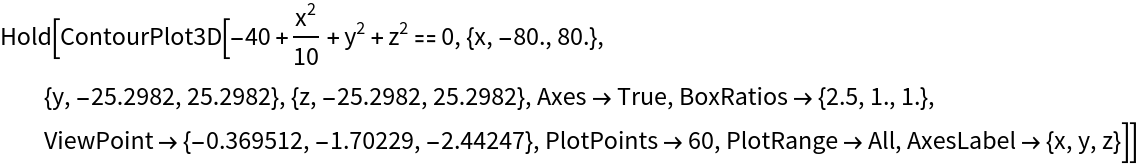Now deploy that code:

 In:=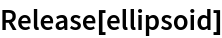Out=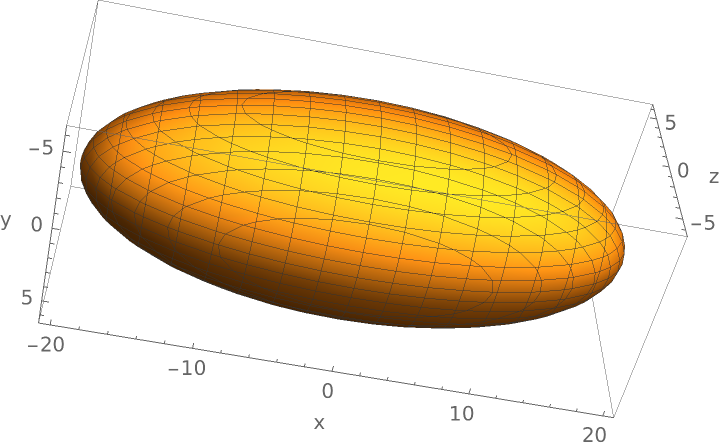Plot a paraboloid:

 In:=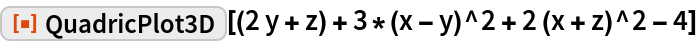Out=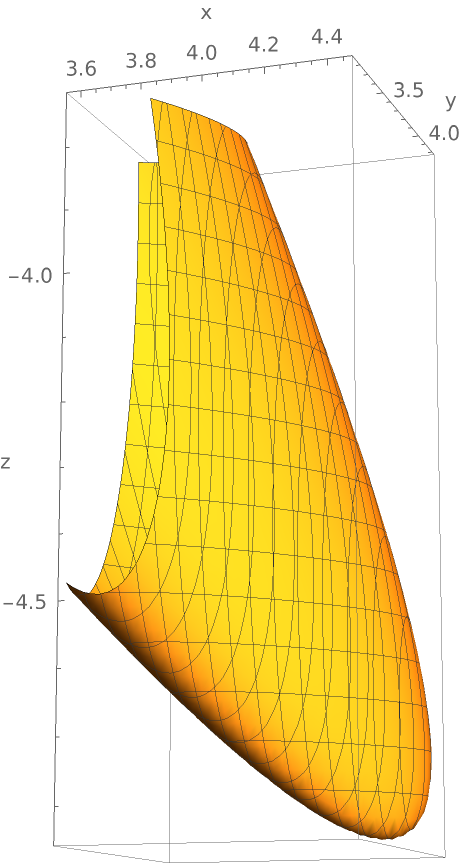### Scope

Plot a cone:

 In:=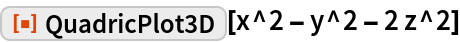Out=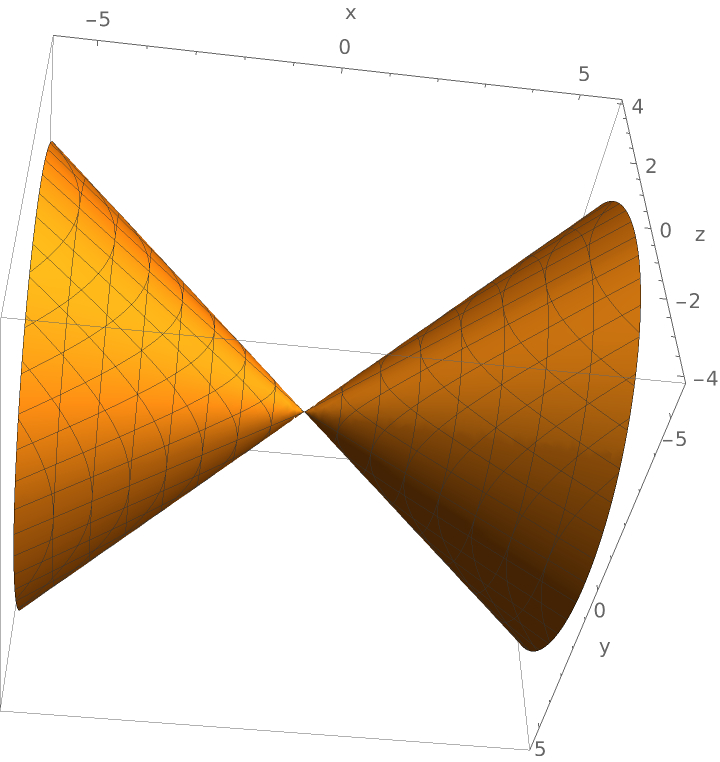Plot a pair of intersecting planes:

 In:=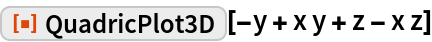Out=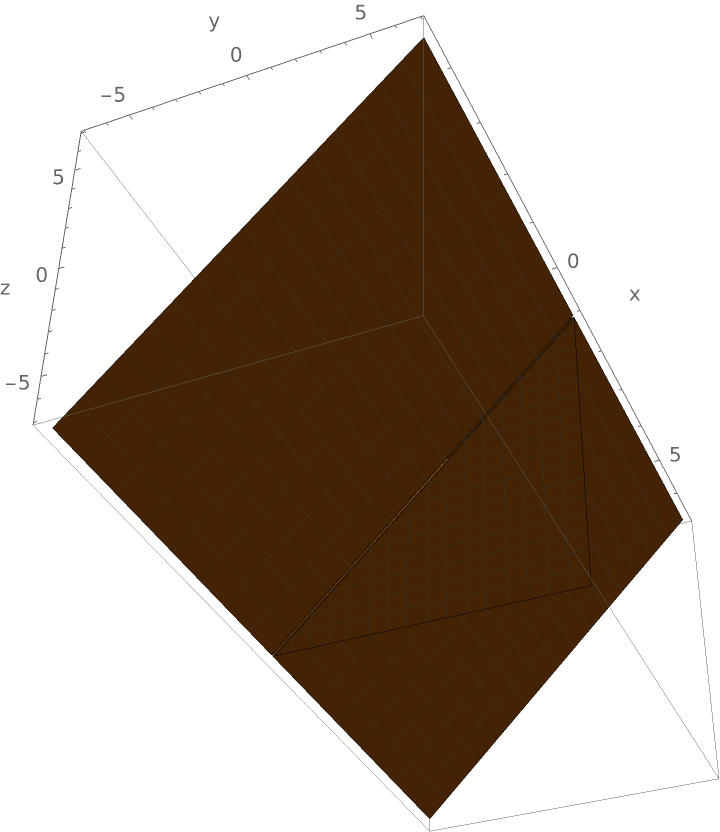These are intersecting planes because the polynomial factors:

 In:=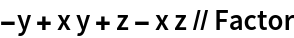Out=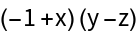Plot a parabolic cylinder:

 In:=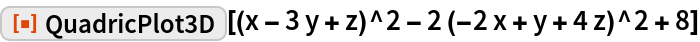Out=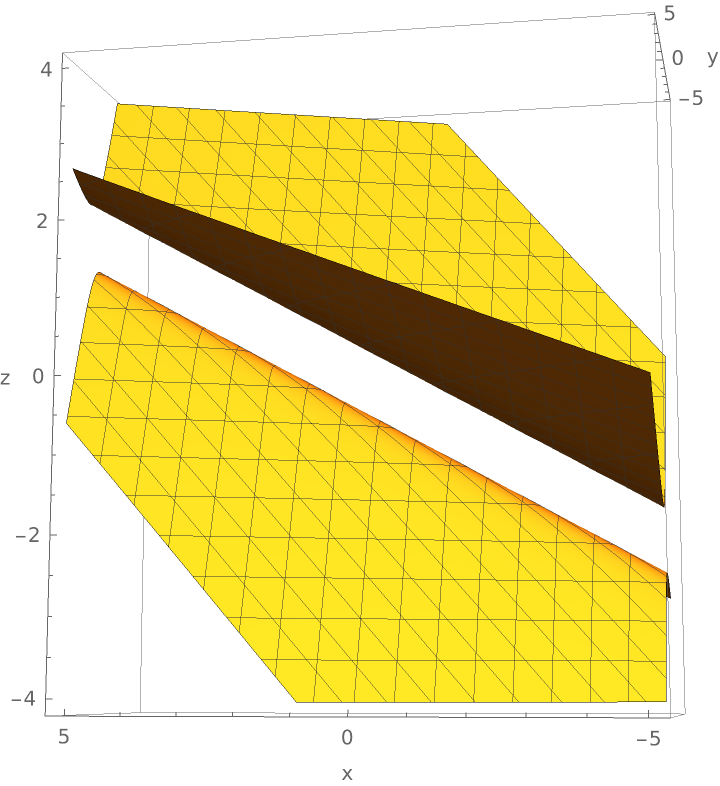In:=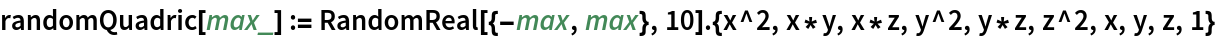In:=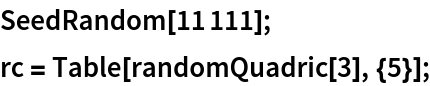In:=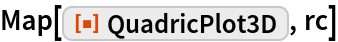Out=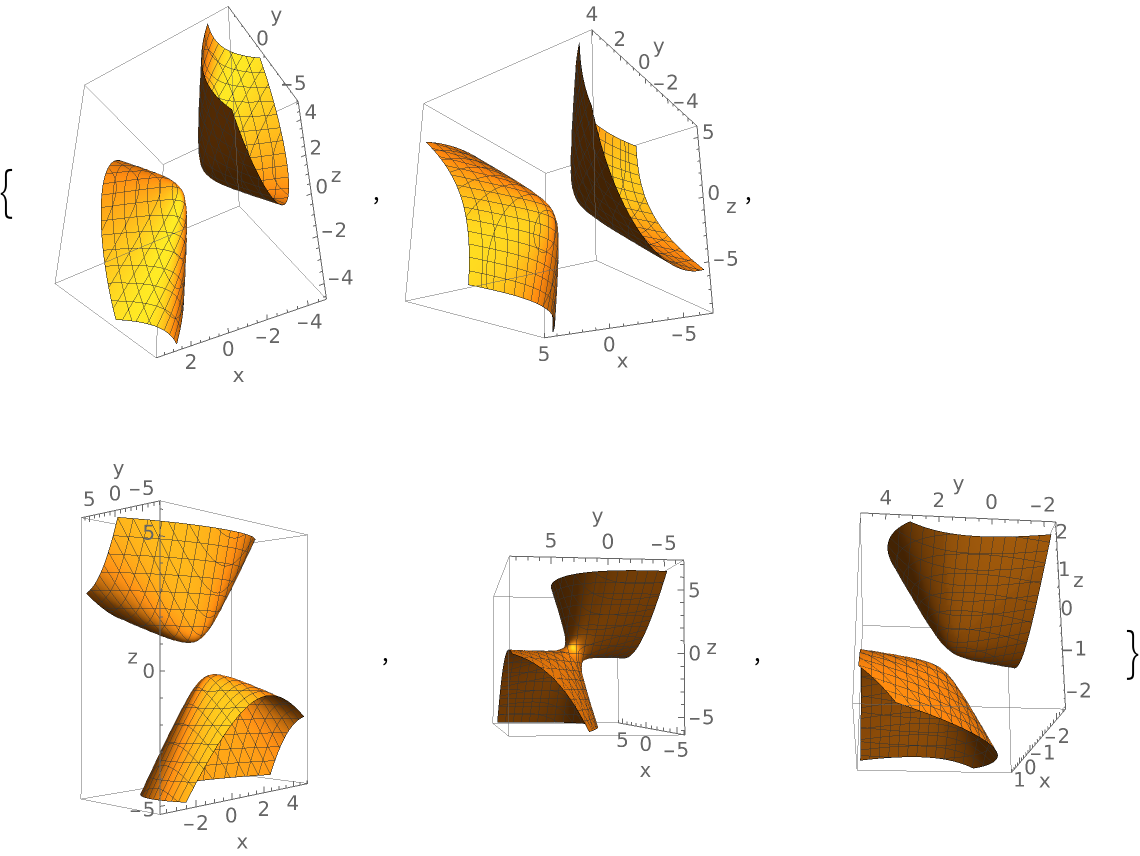### Possible Issues

QuadricPlot3D will return unevaluated if the argument is not discernibly a polynomial of total degree 2 in three variables:

 In:=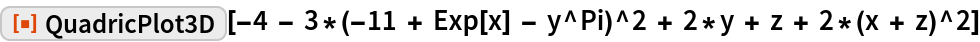Out=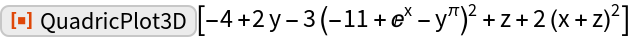For some quadrics, e.g. “thin” hyperboloids of one sheet, QuadricPlot3D may show a view that obscures a part of the saddle:

 In:=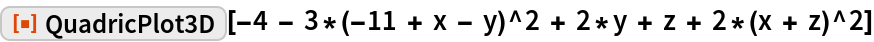Out=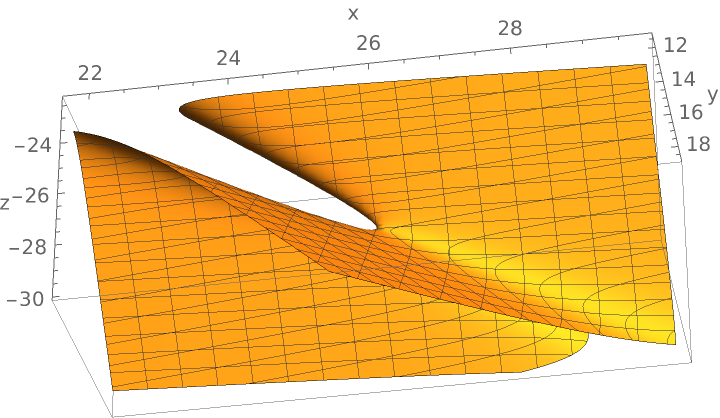One can get different views simply by rotating using the mouse: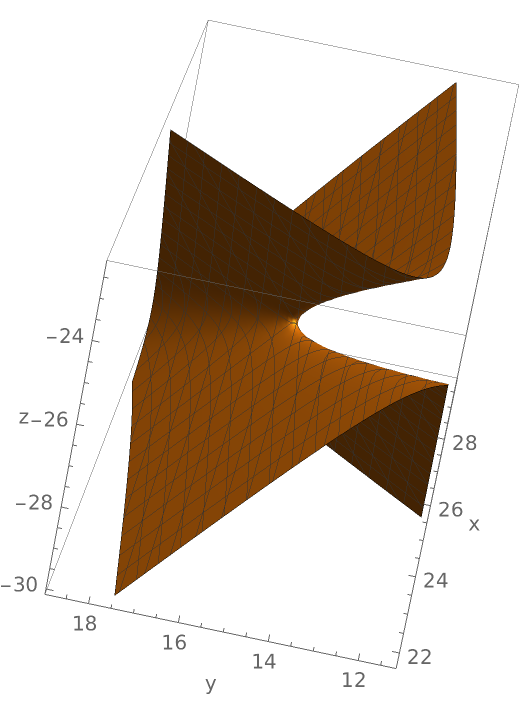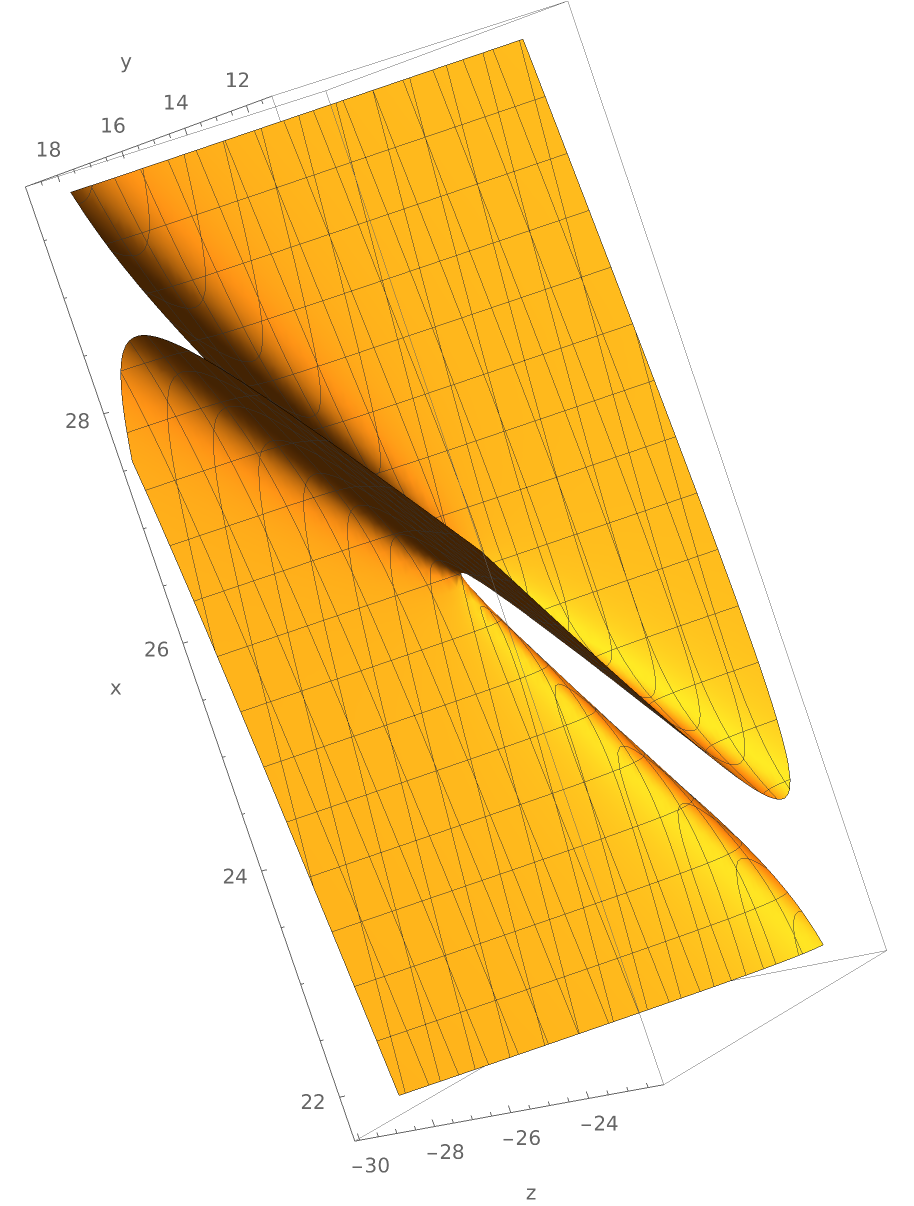3D graphic objects can be large:

 In:=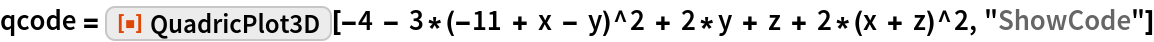Out=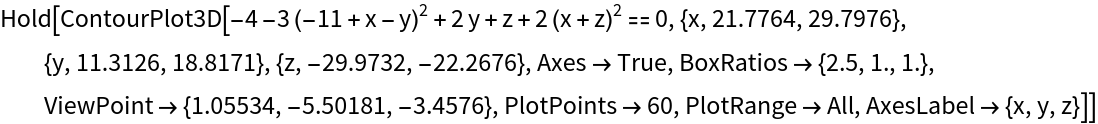In:=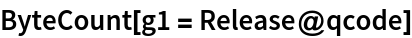Out=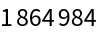One can reduce this considerably by rasterizing the 3D graphic object:

 In:=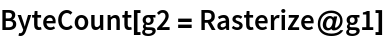Out=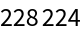There is good fidelity in the rasterized image (though it can no longer be rotated):

 In:=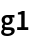Out=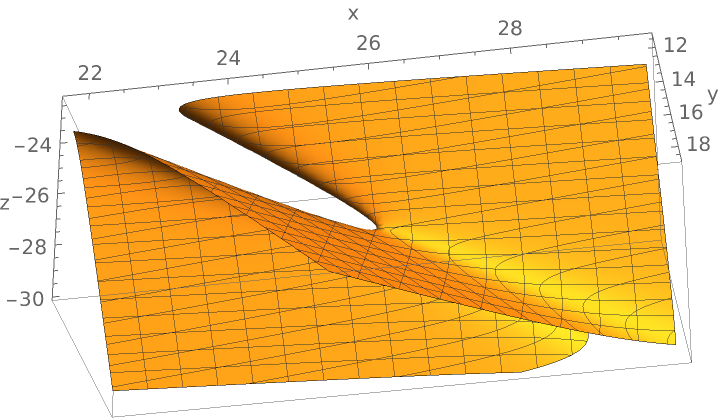In:=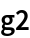Out=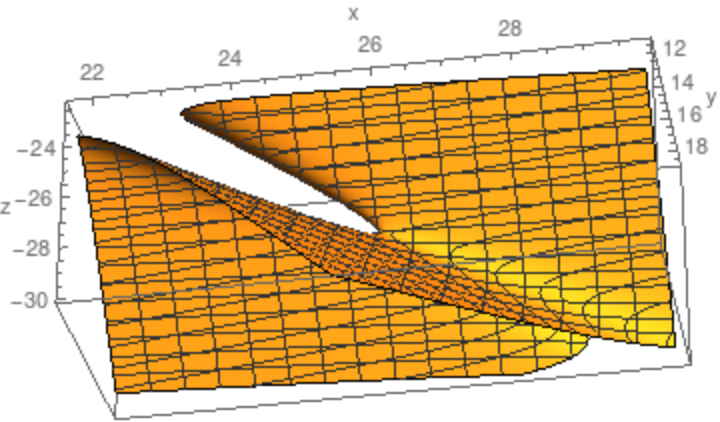## Requirements

Wolfram Language 11.3 (March 2018) or above